## Register Now

Lorem ipsum dolor sit amet, consectetur adipiscing elit.Morbi adipiscing gravdio, sit amet suscipit risus ultrices eu.Fusce viverra neque at purus laoreet consequa.Vivamus vulputate posuere nisl quis consequat.

Power Factor is an important aspect in any electrical system. It indicates the quality of design and management of an electrical installation. It determines how effectively incoming supply is used in the electrical system. In this post we will discuss about what is Power Factor, Power Triangle, its Types, PF Corrections (PFC), Applications, Advantages and Disadvantages.

## What is Power Factor (PF)

PF is the cosine of the angular displacement created when the voltage and current are out of phase. It depends on Active (KV) and Apparent (KVA) Power.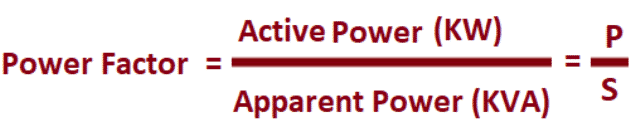Fig. 1 – Equation of PF

### Active Power ‘P’ (KW)

It is the real/working force that drives the electrical equipment that performs the work of heating in a heater, producing torque in a motor etc. It is expressed in kilowatts (KW).

### Reactive Power ‘Q’ (KVAR)

It is the force required by equipment’s like transformers and motors to generate a magnetic field that enables the actual work to be done.  These type of inductive loads dissipate zero energy and draws current which gives the deceptive impression that they actually do dissipate energy.

It often flows back and forth in a circuit. It is expressed in units of Volts-Amps-Reactive(VAR).

### Apparent Power ‘S’ (KVA)

It is the product of a circuit’s voltage and current. The unit to measure it is Volt-Amps (VA).

The value of PF will range from 0 to 1 irrespective of the loads. The load may be a single or a number of current consuming equipment.

## What is Power Triangle

The relationship between Active, Reactive and Apparent power is represented as vectors, in the form of a triangle called as “Power Triangle”.

The quantity of Active and Reactive Powers  are represented as horizontal vector and vertical vector respectively. The Apparent Power is the hypotenuse of a right angle triangle formed by connecting the Active (Real) and Reactive Vectors.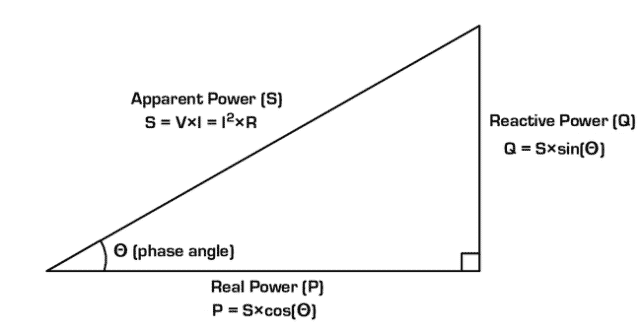Fig. 2 – Power Triangle

The concept of PF can be better understood using a simple analogy as shown in the figure below. Here we are utilizing force as power for better understanding. A man here is dragging a heavy load. His working force or Actual force (Active force) in the forward direction (where he drags his load) is KW. As the load is heavy, the man finds it difficult to drag the load on a perfect horizontal plane and hence his shoulder height adds a little Reactive force, or KVAR. The Apparent force is KVA, which is “vectorial summation” of KVAR and KW.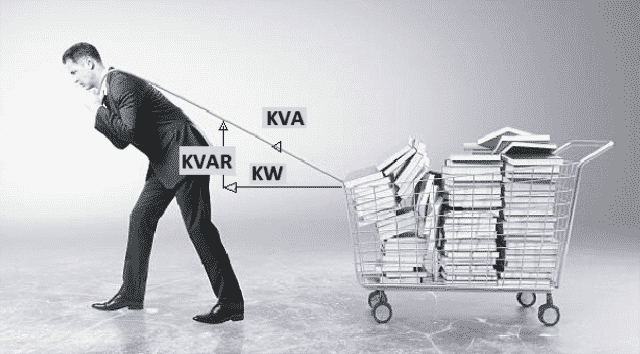Fig. 3 – Example of PF

## Power Factor Types

PF can be differentiated as:

1. Lagging PF

### Lagging PF

The PF of the circuit is said to be ‘Lagging’ when the current lags behind the voltage. It lags in inductive circuits. Induction motors, coils etc are the examples of inductive loads and have lagging PF.

The PF of the circuit is said to be ‘Leading’ when the current leads the voltage (or voltage lags behind the current). The PF leads when the circuit is capacitive. Synchronous condensers, capacitor banks etc are the examples of capacitive loads and have leading PF.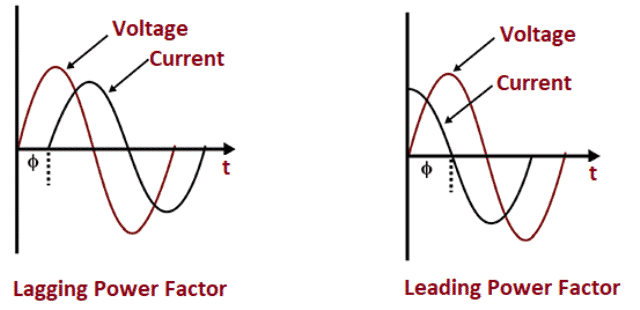Fig. 4 – Lagging and Leading PF

## Power Factor Correction (PFC)

The technique of increasing the PF towards value “1” of an electrical system is called Power Factor Correction (PFC).

Ideally, current and voltage waveforms should be in phase with one another and all the electricity drawn from the AC mains should be utilized effectively. However, there is always a current/ voltage lag that leads to lower PFs due to the reactive components present in a majority of circuits.

Without PFC, the voltage supplies draw current in high-magnitude pulses which has to be smoothed out. This is achieved using PF Correction techniques. Figure below shows the current and voltage waveforms before and after PFC technique.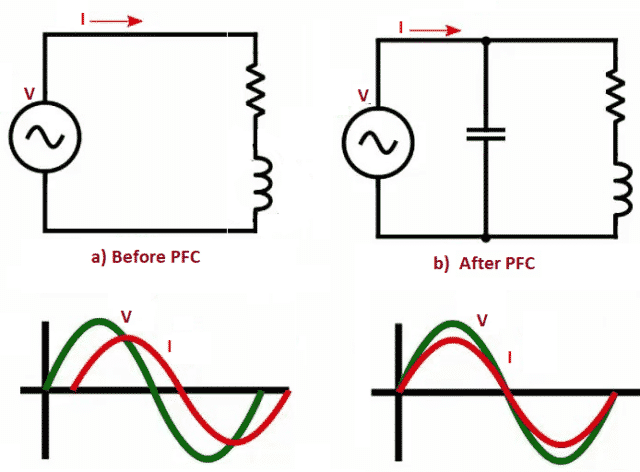Fig. 5 – Current and Voltage Waveforms Before and After PFC

## Applications of Power Factor

The applications of PF are:

• A high PF becomes necessary in a transmission system which reduces transmission losses and improves voltage regulation at the load.
• Its improvisation is important in the systems in which additional load is added and thus system losses is considerably reduced.
• It is increased to make the motors run efficiently and to avoid overheating.

• Reduces electricity charges.
• PF Correction lowers the amount of pollution resulting from releasing carbon emissions in to the atmosphere.
• PFC lowers heat generation in cables, switchgear, transformers and alternators.
• Voltage drop is considerably reduced.

`Also Read: What is Digital Voltmeter - How it Works, Types, Applications, AdvantagesSeries Circuit - Working Principle, Characteristics, Applications, AdvantageThyristor - Working, VI Characteristics, Types, Applications, Advantage & Disadvantage`